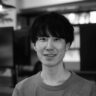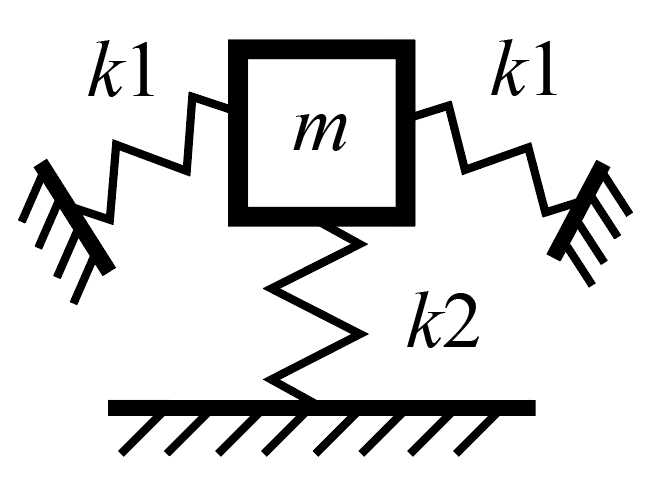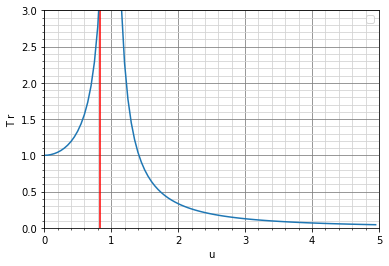# Vibration isolation and its stabilization using the "QZS" structure for quasi-zero stiffness

Quasi-Zero Stiffness structure is known as a technology to solve the trade-off between stiffness, mass, and vibration isolation introduced in the previous article. A search for "Quasi-Zero Stiffness" will turn up several papers (e.g., , , ), so I will give an overview and introduce the solution in NatureArchitects.Hiroaki Natsume

2022,09,16 2022,09,16

# 原理についてk_1k_2 の剛性が以下のようになっている場合、系全体としての剛性の和がほぼ0になる部分を作成することでき、それ準0剛性（QZS：Quasi-Zero Stiffness） と呼びます。 原理としてはとても単純なものになっており、重要な点は片方のばねが負剛性となる区間があることです。この構造が実現することによって以下を可能にし、前回の記事で紹介した剛性と質量と防振の間のトレードオフを解決できます。

1. 特定の荷重まではすべてのバネが正の剛性を持つため、高い剛性を持つ
• 防振対象物を支持しても過大な変位が発生しない
2. 一定の荷重になると正剛性のバネと負剛性のバネを組み合わせになり、系全体として擬似的に0剛性になる
• 剛性がほぼ0になることで固有周波数が非常に小さくなり、振動伝達率が0に近づくため、高い防振効果が発揮できる

QZS 構造については安定してこれらの機能つまり、以下の2つを可能にする仕組みや形状を実現するために複数の研究がなされています。

1. 正剛性区間と負剛性区間、および明瞭なそれらの剛性の切り替わり点を持つ k_1
2. 大変形まで線形な剛性を持つ k_2

# 既存の技術

## 例1：Minus K Technology

Minus K Technology では以下のような原理で鉛直剛性と水平剛性を準0にしており、鉛直については上記で紹介した原理を使った準0剛性になっています。 これらはMinus K Technologyのホームページの Technology のページで確認することが出来ますMinus K Technology では防振装置に載荷する質量にもよりますが、上記の挙動を示す部材を以下のように組み合わせることで、 鉛直、水平ともに 1Hz 程度から防振可能なシステムを実現しており、この周波数は通常の構造では防振困難な値です。※各画像の出典は Minus K Technology のHP によります。

## 例2：負剛性を示す単一の部材### 解析このことから想定していた k1 の挙動に非常に近い部材であることが解析からもわかりました。

### 問題点

この形状は k1 のような剛性を出す上でとても有用ですが、初期の不整に弱い問題点があります。その際の解析結果は以下になります。 オレンジが微小な荷重を加えた際の荷重変形関係になります。初期剛性と最後の剛性は一致していますが、中間の負剛性を発揮する区間の剛性が大きく異なっています。 変形図を見てわかるように左右対称に変形が進んでいないことが原因です。# NatureArchitects の QZS 構造

2つの事例を紹介しましたが、それぞれ以下の問題点がありました。

• Minus K Technology の防振システムは非常に高性能ですが、安定して準0剛性を出すためには複数の部材の組み立てが必要
• 組み立てをなくした単一部材で負剛性を発揮する波状梁は安定して負剛性を出すことが困難

これら二つの事項に対して弊社では、コンプライアントメカニズムを使って組み立てを極力なくした QZS 構造を発明しました。 （特許出願済み）

## 形状

1. 波状梁の中央部は鉛直変位の自由度を持つ
2. 波状梁の中央部は鉛直変位の以外の自由度は持たない
• つまり鉛直変位以外は高い剛性を持つ
3. 波状梁の中央部は回転自由度を持たない
• つまり高い回転剛性を持つ

これらの条件を満たすコンプライアントメカニズムとして、弊社でスライダー構造と呼んでいる以下の剛体と梁からなる形状があります。 この形状は図のように右端を固定した状態で図中赤矢印のように中央のA部を鉛直に動かすときれいに鉛直にのみ変形し、A部は回転や水平方向の変形に対して非常に高い剛性を持っています。 そのため今回のケースで必要とする上記3つの条件に適したコンプライアントメカニズムになっています。

ここでは割愛しますが、興味のある方は実際に上記のような挙動になるのか解析や実験で試してみてください。## 実験

### 実験の様子

#### 重りなしの状態での振動

この状態では想定した0剛性になっていないため、振動が増幅して機構の上面に伝達されています。

### 実験結果

この構造の重りを載せる前の初期剛性は約6N/mm、載せた重りは1kg なので、そのまま線形な剛性をもつ場合は固有周波数は12Hz程度となります。 10Hzで加振しているため振動数比は u=10/12 \fallingdotseq 0.83 となり前回の記事で紹介した振動伝達率 Tr のグラフにその値をプロットしたものが以下です。この結果を見るとほぼ共振する箇所に振動数比 u が来ているため、防振効果が発揮されず振動は非常に増幅して伝達されるはずです。ですが、1kg載荷時に準ゼロ剛性になるように設計しているため、上記の動画のように振動が増幅せず減衰しており、防振できていることが確認できます。

#### 問題点

QZSの効果によって防振効果を発揮していることが確認できましたが、一方で揺らし始めや振動可視化のための水を置いた際にふわふわと振動していることが実験動画からわかります。

この振動は加振による強制振動ではなく、手で振れたことによって一瞬荷重がかかり、それによって発生した自由振動です。 減衰が小さいので振動が手で押さえるまで止まらずにいます。

この問題は、試験体を減衰が小さいアルミ材料のみで作成し、別途減衰部材を設けていないため起きたと想定しており、強制振動以外での安定性が改善すべき点だとわかりました。

# まとめ

どのように減衰を付与してよりロバストな防振装置とするかは今後の課題ですが、剛性、質量、防振のトレードオフを突破し、高い鉛直支持力を持ち、かつ低周波数でも防振可能にする QZS 構造の紹介でした。

# 参考資料

1. HaiguiFan et al. (2020). Design of metastructures with quasi-zero dynamic stiffness for vibration isolation. Composite Structures, 243
2. Mohan Zhao (2016). Is the negative equivalent stiffness of a system possible? PhysicsEducation, 51
3. A.Carrella et al. (2006). Static analysis of a passive vibration isolator with quasi-zero-stiffness characteristic. Journal of Sound and Vibration, 301

# About the Mechanism

Consider a system of three springs supporting one mass point as follows. The stiffness of the diagonal spring is k_1 and that of the vertical spring is k_2.If the stiffnesses of k_1 and k_2 are as follows, it is possible to create a region where the sum of the stiffnesses of the entire system is approximately zero, thereby achieving a quasi-zero stiffness, which is called QZS. The mechanism is very simple, and the important point is that there is a region where one of the springs has negative stiffness.This structure allows for the following and solves the trade-off between stiffness, mass and vibration isolation described in the previous article

1. high stiffness because all springs have positive stiffness up to a specific load
• Even if the object to be isolated from vibration is supported, excessive displacement will not occur.
2. At a specific load, a spring with positive stiffness is combined with a spring with negative stiffness, and the system as a whole becomes quasi-zero stiffness.
• Since the natural frequency becomes very small and the vibration transmission coefficient approaches zero due to the quasi zero stiffness, a high vibration isolation effect can be achieved.

There are several studies on the QZS structure to realize a mechanism and geometry that can stably realize these functions, namely, the following two.

1. k_1 with positive and negative stiffness intervals and their distinct stiffness switching points
2. Linear stiffness up to large deformations k_2.

# Known technologies

The above load-deformation relationship shows that it is theoretically possible to achieve high support but quasi-zero stiffness. This section describes how this is realized in the actual member.

## CASE 1 : Minus K Technology

Structures that achieve quasi-zero stiffness by combining negative and positive stiffness have already been commercialized and are well known by a company called Minus K Technology.

The above video shows that the wine glasses and hula dancers on the vibration isolator are not shaking at all.

Minus K Technology uses the following mechanisms to achieve quasi-zero vertical and horizontal stiffness, and for vertical, quasi-zero stiffness using the mechanisms described above. These can be found on the Minus K Technology website at. Technology

Vertical directionhorizontal directionAlthough it depends on the mass loaded on the vibration isolator, Minus K Technology has realized a system that can isolate vibration from about 1 Hz both vertically and horizontally by combining components that exhibit the above behavior as shown below, a frequency that is difficult to achieve with ordinary structures.※The source of each image is from Minus K Technology's website.

## CASE 2 : Single member showing negative stiffness

You may be able to imagine an actual member for k2 that is stable to large deformation and exhibits linear stiffness (e.g., a coil spring), but you may be wondering if there is a single member that exhibits zigzagging stiffness like k1 without assembly like Minus K Technology I think so. The answer is known to be a corrugated beam, as shown below.

The following corrugated beam is analyzed with ANSYS, a finite element CAE software, to confirm that negative stiffness is produced.### Analysis

Large deformation analysis is performed with the following boundary conditions. Loads are applied as forced displacements with horizontal free and restrained only in the vertical direction.The following is a model diagram of the load-deformation relationships and deformations obtained from the analysis performed. The model is shown at 1x magnification.There is a slight nonlinearity in stiffness around the range where the deformation exceeds 12 mm, but both positive and negative stiffnesses are nicely linear, and there is a clear stiffness switching point.

The analysis showed that the member is very close to the assumed k1 behavior.

### Problem

This shape is very useful in producing stiffness like k1, but has the problem of being vulnerable to initial irregularities.

Here is a sample. Assuming that the load was not given exactly vertically, the same analysis as before is performed with a very small load in the axial direction of the beam, as shown below.The results of the analysis are shown below. Orange shows the load-deformation relationship when a small load is applied. The initial and final stiffnesses are in a similar state, but the stiffness of the middle section, which exhibits negative stiffness, is significantly different. This is due to the fact that the deformation is not symmetrical as can be seen in the deformation diagram.The negative stiffness of a corrugated beam is caused by the effect of axial forces entering the beam as it is deformed. Therefore, if the beam is not deformed symmetrically, the axial force is not introduced into the beam properly and the expected negative stiffness cannot be obtained.

# NatureArchitects' QZS Structure

Two case studies were presented, each with the following problems.

• Minus K Technology's vibration isolation system is very high performance, but requires assembly of multiple members to achieve stable quasi-zero stiffness
• Corrugated beams, which provide negative stiffness with a single member that eliminates assembly, are difficult to achieve stable negative stiffness.

To address both of these issues, we have invented the QZS structure, which uses a compliant mechanism to minimize assembly. (Patent pending)

## Geometry

In order to achieve the expected negative stiffness, the corrugated beam must have a symmetrical and clean vertical displacement in the center. If we break this down a bit more, we find that the following three conditions are necessary for stabilization.

1. the center of a corrugated beam has a degree of freedom for vertical displacement
2. the center of the corrugated beam has no degrees of freedom except for vertical displacement
• In other words, it has high stiffness except for vertical displacement.
3. the center of the corrugated beam has no rotational degrees of freedom
• In other words, it has high rotational stiffness.

A compliant mechanism that satisfies these conditions is what we call a slider structure, which consists of the following rigid body and beams. As shown in the figure, when the right end is fixed and the center part A is moved vertically as indicated by the red arrow in the figure, it is deformed exactly only vertically, and part A has very high rigidity against rotation and horizontal deformation. Therefore, it is a compliant mechanism suitable for the above three conditions required in this case.

The details are omitted here, but those who are interested should try analysis and experiment to see if the above behavior actually occurs.By combining the above slider structure and corrugated beams as follows, we were able to create a structure that restrains the center of the corrugated beam with high stiffness except for vertical displacement.## Experiment

We created a prototype of the above component and confirmed that it actually exhibits the expected vibration isolation behavior.

We not only calculate and design the shape, but also have a simple in-house experimental facility that allows us to actually create and test the calculated shape.

The test piece was created by a wire electrical discharge machining company.

### Behavior in experiments

This video shows an experiment using a vibration table. Both are vibrated at 10 Hz, with the only difference being the presence or absence of weights.

#### Vibration without weight

In this state, vibration is amplified and transmitted to the top surface of the mechanism because it is not at the assumed 0 stiffness.

#### Vibration with weight loaded and in QZS state

The addition of the load by the weights has resulted in a quasi-zero stiffness state, which reduces the shaking of the water surface on top of the member relative to the surface connected to the shaking table below, i.e., the vibration isolation effect is achieved.

### Results of experiments

Experiments also confirmed that quasi-zero stiffness was achieved. Although no video is available, the same vibration isolation effect was obtained even when the position of the mass to be placed was changed to the edge on the top plate (i.e., no clean vertical load was applied).

The initial stiffness of this structure before loading the weight is about 6N/mm, and the loaded weight is 1kg, so the natural frequency is about 12Hz for linear stiffness as it is. The vibration frequency ratio is u=10/12 \fallingdotseq 0.83, and the value is plotted on the graph of the vibration transmission coefficient Tr introduced in the previous article. The result shows that the vibration frequency ratio u is almost at the resonance point, which means that the vibration isolation effect is not effective and the vibration should be amplified and transmitted very much.However, since the design is such that the quasi-zero stiffness is reached when 1 kg is loaded, the vibration is not amplified and is damped as shown in the above video, confirming that the vibration isolation is achieved.

#### Future work

The experimental video shows that the QZS has an anti-vibration effect, but on the other hand, it vibrates softly at the beginning of shaking and when water is placed on it to visualize the vibration.

This vibration is not a forced vibration caused by excitation, but a free vibration caused by a momentary load applied by hand shaking. Since the damping is small, the vibration does not stop until it is supported by the hand.

It is assumed that this problem occurred because the test specimen was made only of aluminum material with low damping and no separate damping member was provided, and it was found that stability other than forced vibration should be improved.

# Summary

How to add damping to make the vibration isolator more robust remains a challenge for the future, but this was an introduction to the QZS structure, which breaks through the trade-off between stiffness, mass, and vibration isolation to provide high vertical support and vibration isolation even at low frequencies.

We believe that we were able to introduce part of our design process to improve the performance of existing components by identifying the degrees of freedom necessary to improve the functionality of known components and combining them with geometries (metamaterials, compliant mechanisms, etc.) that allow appropriate control of the degrees of freedom.

# Reference

1. HaiguiFan et al. (2020). Design of metastructures with quasi-zero dynamic stiffness for vibration isolation. Composite Structures, 243
2. Mohan Zhao (2016). Is the negative equivalent stiffness of a system possible? PhysicsEducation, 51
3. A.Carrella et al. (2006). Static analysis of a passive vibration isolator with quasi-zero-stiffness characteristic. Journal of Sound and Vibration, 301

Nature Architects株式会社

Nature Architects, Inc.

CEO:Taisuke Ohsima

ADDRESS:Apartment Calm 3D, 8-4-7, Akasaka, Tokyo, 107-0052, Japan

Funded:May, 2017

お問い合わせ:info@nature-architects.com

CONTACT:info@nature-architects.com